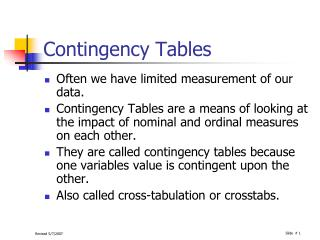DownloadDownload PresentationContingency Tables

# Contingency Tables

Télécharger la présentation## Contingency Tables

- - - - - - - - - - - - - - - - - - - - - - - - - - - E N D - - - - - - - - - - - - - - - - - - - - - - - - - - -
##### Presentation Transcript

1. Contingency Tables • Often we have limited measurement of our data. • Contingency Tables are a means of looking at the impact of nominal and ordinal measures on each other. • They are called contingency tables because one variables value is contingent upon the other. • Also called cross-tabulation or crosstabs.

2. Contingency Tables • The procedure is quite simple and intuitively appealing • Construct a table with the independent variable across the top and the dependent variable on the side • This works fairly well for low numbers of categories (r,c < 6 or so)

3. Contingency Tables An example • Presidents are often suspected of using military force to enhance their popularity. • What do you suppose the data actually look like? • Any conjectures • Let’s categorize presidents as using force,or not, and as having popularity above and below 50% • Are there definition problems here? • Which is independent and which is dependent?

4. Contingency Tables

5. Measures of Independence • Are the variables actually contingent upon each other? • Is the use of force contingent upon the president’s level of popularity? • We would like to know if these variables are independent of each other, or does the use of force actually depend upon the level of approval that the president have at that time?

6. 2 Test of Independence • The 2 Test of Independence gives us a test of statistical significance. • It is accomplished by comparing the actual observed values to those you would expect to see if the two variables are independent.

7. 2 Test of Independence • Formula • Where

8. Chi-Square Table (2)

9. Interpreting the 2 • The Table gives us a 2 of 5.55 with 1 degree of freedom [d.f. = (r-1)*(c-1)] • The critical value of 2 with 1 degree of freedom is 3.84 (see 2 Table) • We therefore conclude that Presidential popularity and use of force are related. • We technically “reject the null hypothesis that Presidential popularity and use of force are independent.” • Note: 2 is influenced by sample size. • It ranges from 0.0 to .

10. Corrected 2 measures • Small tables have slightly biased measures of 2 • If there are cell frequencies that are low, then there are some adjustments to make that correct the probability estimates that 2 provides.

11. Yate’s Corrected 2 • For use with a 2x2 table with low cell frequencies (5<n<10) • If there are any cell frequencies < 5, the 2 is invalid. • Use Fisher’s Exact Test

12. Measures of Association • Not only do we want to see whether the variables of a cross-tabulation are independent, we often want to see if the relationship is a strong or weak one. • To do this, we use what are referred to as measures of association. • The level of measurement determines what measure of association we might use.

13. Measures of Association • We group them according to whether the variables are nominal or ordinal. • If one variable is nominal, use nominal measures. • If both are ordinal, use an ordinal measure. • If either is interval, generally we use a different statistical design.

14. Measures based on 2 • Contingency Coefficient • Kramer’s V

15. Yule’s Q • May be used on any 2x2 table, nominal or ordinal • If we define out table with cell counts as • Yule’s Q is calculated as: • Q ranges from 0 to 1.0 • Q compares concordant pairs to discordant pairs

16. Phi

17. Gamma • Will equal 1.0 if any cell is empty

18. Lambda • Asymmetric measure of association • Calculation depends on whether the column variable or the row variable is independent

19. Ordinal Measures • Goodman & Kruskal’s Gamma • For Ordinal x Ordinal tables • May also be used if one of the variables is a nominal dichotomy

20. Lambda • Asymmetrioc

21. Tau-b & Tau-c • Similar to Gamma • If r=c, use tau-b; if r<>c, use tau-c